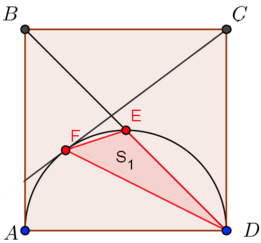# Square, Triangle, And Semicircle Living In HarmonyIn the figure above:

• The square $ABCD$ has area 12.25.

• $E$ is the intersection of $BD$ and the semicircle of diameter $AD.$

• $CF$ is a segment drawn from $C$ and tangent to the semicircle at $F.$

Calculate the area of the triangle $DEF.$ Give your answer to three decimal places.

×